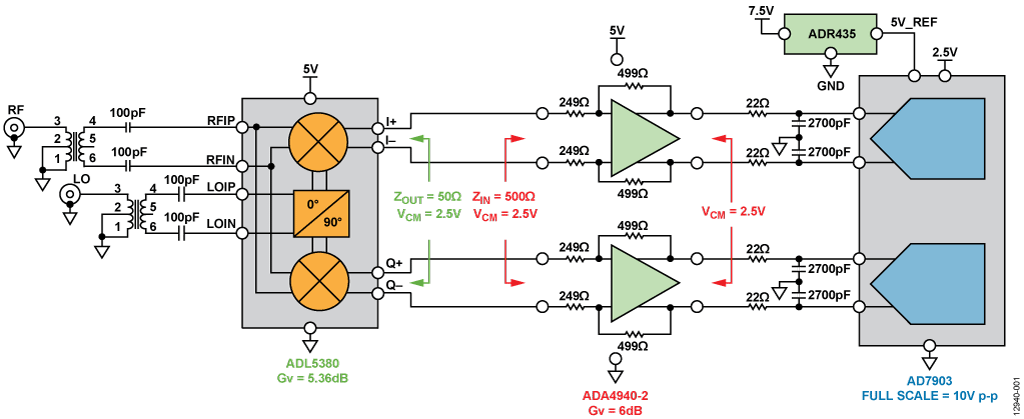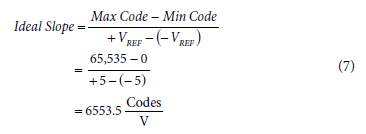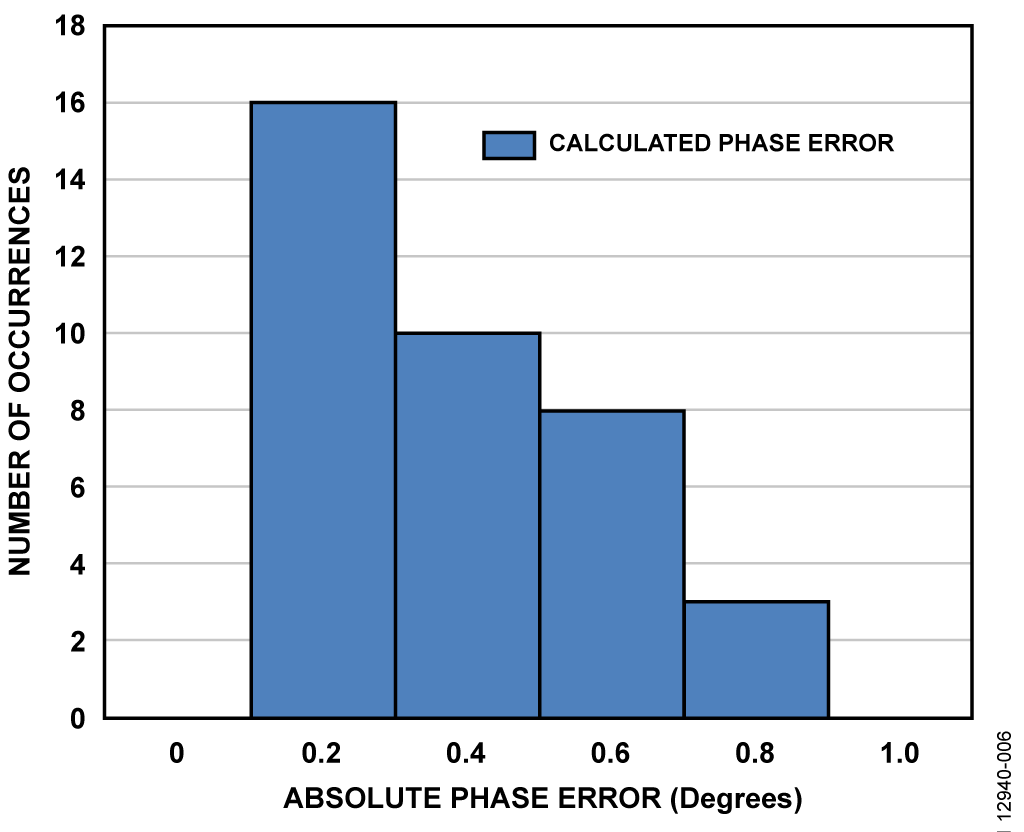Оценочные платы \$108.90## Ресурсы проектирования

### Файлы проектирования и интеграции

• Schematic
• Bill of Materials
• Gerber Files
• Assembly Drawing

### Оценочные платы

Буква "Z" в наименовании компонента указывает на соответствие требованиям RoHS. Отмеченные платы нужны для оценки данной схемы

• EVAL-SDP-CB1Z (\$108.90) Eval Control Board

### Драйверы устройств

ПО (код на С и/или FPGA) для связи с цифровым интерфейсом компонента.

## Особенности и преимущества

• Precise Magnitude and Phase Measurements to 6GHz
• Small PCB Footprint

## Функции и преимущества схемы

The circuit shown in Figure 1 precisely converts a 400 MHz to 6 GHz RF input signal to its corresponding digital magnitude and digital phase. The signal chain achieves 0° to 360° of phase measurement with 1° of accuracy at 900 MHz. The circuit uses a high performance quadrature demodulator, a dual differential amplifier, and a dual differential 16-bit, 1 MSPS successive approximation analog-to-digital converter (SAR ADC).Figure 1. Simplified Receiver Subsystem for Magnitude and Phase Measurements (All Connections and Decoupling Not Shown)

## Описание схемы

A quadrature demodulator provides an in-phase (I) signal and a quadrature (Q) signal that are exactly 90° out of phase. The I and Q signals are vector quantities; therefore, the amplitude and phase shift of the received signal can be calculated using trigonometric identities, as shown in Figure 2. The local oscillator (LO) input is the original transmitted signal and the RF input is the received signal. The demodulator generates a sum and difference term. Both the RF and LO signals are at the exact same frequency, ωLO = ωRF, and therefore the high frequency sum term is filtered, while the difference term resides at dc. The received signal has a different phase (φRF) than that of the transmitted signal (φLO), and this phase shift can be represented as φLO − φRF.

A real-world I/Q demodulator has many imperfections, including quadrature phase error, gain imbalance, and LO to RF leakage, all of which can degrade the quality of the demodulated signal. To select a demodulator, first determine the requirements for RF input frequency range, amplitude accuracy, and phase accuracy. Powered from a single 5 V supply, the ADL5380 demodulator accepts RF or IF input frequencies from 400 MHz to 6 GHz, making it ideal for the receiver signal chain. Configured to provide a 5.36 dB voltage conversion gain, the differential I and Q outputs of the ADL5380 can drive a 2.5 V p-p differential signal into a 500 Ω load. Its 10.9 dB noise figure (NF), 11.6 dBm firstorder intercept (IP1), and 29.7 dBm third-order intercept (IP3) t 900 MHz provide outstanding dynamic range; and its 0.07 dB amplitude balance and 0.2° phase balance achieve excellent demodulation accuracy. Manufactured using an advanced SiGe bipolar process, the ADL5380 is available in a tiny 4 mm × 4 mm, 24-lead LFCSP package.

The excellent dynamic performance and adjustable output common-mode voltage of the ADA4940-2 fully differential dual amplifier make it ideal for driving high resolution, dual SAR ADCs. Powered from a single 5 V supply, the ADA4940-2 provides ±5 V differential outputs with a 2.5 V common-mode voltage. Configured to provide a gain of 2 (6 dB), it drives the ADC inputs to full-scale. The RC filter (22 Ω/2.7 nF) limits the noise and reduces the kickback coming from the capacitive digital-toanalog converter (DAC) at the ADC input. Manufactured using a proprietary SiGe complementary bipolar process, the ADA4940-2 is available in a tiny 4 mm × 4 mm, 24-lead LFCSP package.

The AD7903 dual 16-bit, 1 MSPS SAR ADC offers excellent precision, with ±0.006% FS gain error and ±0.015 mV offset error. Operating from a single 2.5 V power supply, the AD7903 dissipates only 12 mW at 1 MSPS. The main goal of using a high resolution ADC is to achieve ±1° phase accuracy, especially when the input signal has a small dc amplitude. The 5 V reference required by the ADC is generated by the ADR435 low noise reference.

## Основные варианты исполнения

The frequency range of the circuit can be extended to lower frequencies by using the ADL5387 30 MHz to 2 GHz quadrature demodulator.

Depending on the specific application, the amplifier between the demodulator and ADC may or may not be necessary. The ADL5380 can interface directly to the AD7903 because the common-mode voltages of both devices are compatible. If using an alternative ADC with a common-mode voltage that is not within the range of the demodulator, an amplifier is necessary to achieve the level translation with minimal power loss.

## Оценка параметров и тестирование схемы

As shown in Figure 3, the receiver subsystem is implemented using the ADL5380-EVALZ, ADA4940-2ACP-EBZ, EVALAD7903SDZ, and EVAL-SDP-CB1Z evaluation kits. These circuit components are optimized for interconnection in the subsystem. Two high frequency, phase-locked input sources provide the RF and LO input signals.

Table 1 summarizes the input and output voltage levels for each of the components in the receiver subsystem. An 11.6 dBm signal at the RF input of the demodulator produces an input within −1 dB of the ADC full-scale range. Table 1 assumes a 500 Ω load, 5.3573 dB conversion gain, and −4.643 dB power gain for the ADL5380, and 6 dB gain for theADA4940-2. The calibration routine and performance results achieved for this receiver subsystem are discussed in the following sections.

 RF Input ADL5380 Output AD7903 Input +11.6 dBm +6.957 dBm 4.455 V p-p -1.022 dBFS 0 dBm -4.643 dBm 1.172 V p-p -12.622 dBFS -20 dBm -24.643 dBm 0.117 V p-p -32.622 dBFS -40 dBm -44.643 dBm 0.012 V p-p -52.622 dBFS -68 dBm -72.643 dBm 466 µV p-p -80.622 dBFS

The receiver subsystem contains three major error sources: offset, gain, and phase.

The individual differential dc magnitudes of the I and Q channels have sinusoidal relationships with respect to the relative phase of the RF and LO signals. As a result, the ideal dc magnitude of the I and Q channels can be calculated as follows:As the phase moves through the polar grid, some locations ideally produce the same voltage. For example, the voltage on the I (cosine) channel should be identical with phase shifts of +90° or −90°. However, a constant phase shift error, independent of the relative phase of RF and LO, causes the subsystem channel to generate different results for input phases that should produce the same dc magnitude. This is illustrated in Figure 4 and Figure 5, where two different output codes are generated when the input should be at 0 V. In this case, the −37° phase shift is much larger than expected in a real-world system containing phase-locked loops. The result is +90° actually appearing as +53°, and −90° as −127°.

 Input Phase RF to LO Average I Channel Output Code Average Q Channel Output Code I Channel Voltage Q Channel Voltage Measured Phase Measured Receiver Subsystem Phase Shift -180° -5851.294 +4524.038 -0.893 V +0.690 V +142.29° -37.71° -90° -4471.731 -5842.293 -0.682 V -0.891 V -127.43° -37.43° 0° +5909.982 -4396.769 +0.902 V -0.671 V -36.65° -36.65° +90° +4470.072 +5858.444 +0.682 V +0.894 V +52.66° -37.34° +180° -5924.423 +4429.286 -0.904 V +0.676 V +143.22° -36.78°

Results were gathered in 10° steps from −180° to +180°, with the uncorrected data generating the elliptical shapes shown in Figure 4 and Figure 5. This error can be accounted for by determining the amount of additional phase shift present in the system. Table 2 shows that the system phase shift error is constant throughout the transfer function.

### System Phase Error Calibration

With a step size of 10°, the average measured phase shift error was −37.32° for the system shown in Figure 3. With this additional phase shift known, the adjusted subsystem dc voltages can now be calculated. The variable φPHASE_SHIFT is defined as the average observed additional system phase shift. The dc voltage generated in the phase-compensated signal chain can be computed asEquation 5 and Equation 6 provide the target input voltage for a given phase setting. The subsystem has now been linearized, and the offset error and gain error can now be corrected. The linearized I and Q channel results can also be seen in Figure 4 and Figure 5. A linear regression on the data sets generates the best fit line shown in the figures. This line is the measured subsystem transfer function for each conversion signal chain.

### System Offset and Gain Error Calibration

The offset of each signal chain within the receiver subsystem is ideally 0 LSB; however, the measured offsets were −12.546 LSB and +22.599 LSB for the I and Q channels, respectively. The slope of the best fit line represents the slope of the subsystem. The ideal subsystem slope can be calculated asThe results in Figure 4 and Figure 5 show that that measured slopes were 6315.5 and 6273.1 for the I and Q channels, respectively. These slopes must be adjusted to correct the system gain error. Correcting for gain error and offset error ensures that the signal magnitude computed using Equation 1 matches the ideal signal magnitude. The offset correction is the opposite of the measured offset error:The gain error correction coefficient isThe received conversion result can be corrected byThe calibrated dc input voltage of the subsystem is calculated asUse Equation 11 on both the I and Q channels to compute the perceived analog input voltage for each subsystem signal chain. These fully adjusted I and Q channel voltages are used to compute the RF signal amplitude as defined by the individual dc signal magnitudes. To evaluate the accuracy of the full calibration routine, convert the collected results to ideal subsystem voltages produced at the output of the demodulator as if no phase shift error were present; multiply the average dc magnitude computed previously by the sinusoidal fraction of the measured phase at each trial with the computed phase shift error removed. The calculation is as follows:where:

φPHASE_SHIFT is the phase error previously computed. Average Post Calibration Magnitude is the dc magnitude result from Equation 1 that has been compensated for offset error and gain error.

Table 3 shows the results of the calibration routine at various target phase inputs for the 0 dBm RF input amplitude case. The calculations performed in Equation 12 and Equation 13 are the correction factors to be built into any system intended to sense phase and magnitude in the manner described in this circuit note.

 Target Phase I Channel Fully Corrected Input Voltage Q Channel Fully Corrected Input Voltage Fully Corrected Phase Result Absolute Measured PhaseError -180° -1.172 V +0.00789 V -180.386° 0.386° -90° -0.00218 V -1.172 V -90.107° 0.107° 0° +1.172 V +0.0138 V +0.677° 0.676° +90° +0.000409 V +1.171 V +89.98° 0.020° +180° -1.172 V -0.0111 V +180.542° 0.541°

Figure 6 is a histogram of the measured absolute phase error showing better than 1° accuracy for every 10° step from −180° to +180°.Figure 6. Measured Absolute Phase Error Histogram for 0 dBm Input Level with 10° Phase Steps

For accurate phase measurements at any given input level, the perceived phase shift error (φPHASE_SHIFT) of RF relative to LO must be constant. If the measured phase shift error begins to change as a function of the target phase step (θTARGET) or amplitude, the calibration routine presented in this section begins to lose accuracy. Evaluation results at room temperature show that the phase shift error is relatively constant for RF amplitudes ranging from a maximum of 11.6 dBm to approximately −20 dBm at 900 MHz.

Figure 7 shows the dynamic range of the receiver subsystem along with the corresponding amplitude-induced additional phase error. As the input amplitude decreases past −20 dBm, the phase error calibration accuracy begins to degrade. The system user must determine the acceptable level of signal chain error to determine the minimum acceptable signal magnitude.

The results shown in Figure 7 were collected with a 5 V ADC reference. The magnitude of the ADC reference can be reduced, providing a smaller quantization level for the system, which provides an incremental improvement in phase error accuracy for small signals but increases the chance for system saturation. To increase system dynamic range, another option is to implement an oversampling scheme that increases the noise-free bit resolution of the ADC. Every doubling in samples averaged provides a ½ LSB increase in system resolution. The oversampling ratio for a given resolution increase is calculated as follows:where N is the number of bits increase.

Oversampling reaches a point of diminishing returns when the noise amplitude is no longer sufficient to randomly change the ADC output code from sample to sample. At this point, the effective resolution of the system can no longer be increased. The bandwidth reduction from oversampling is not a significant concern because the system is measuring signals with a slowly changing magnitude.

The AD7903 evaluation software is available with a calibration routine that allows the user to correct the ADC output results for the three sources of error: phase, gain, and offset. The user must collect uncorrected results with their system to determine the calibration coefficients calculated in this circuit note. Figure 8 shows the Amp/Phase Panel tab of the GUI with the calibration coefficients highlighted. When the coefficients are determined, this tab can also be used to deliver phase and magnitude results from the demodulator. The polar plot provides a visual indication of the observed RF input signal. The amplitude and phase calculations are performed using Equation 1 and Equation 2. The oversampling ratio can be controlled by adjusting the number of samples per capture using the Num Samples drop-down box.

### Equipment Needed

The following equipment are used to evaluate the circuit.

• A Windows® XP, Windows Vista (32-bit), or Windows 7 (32-bit) PC with USB port.
• Two RF signal generators with phase control (such as the R&S SMT06).
• A digital multimeter.
• 5 V and 9 V power supplies.
• The AD7903 evaluation software, used to digitally process the resulting magnitude and phase information.